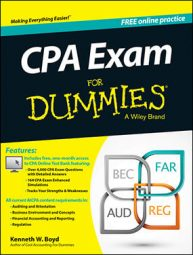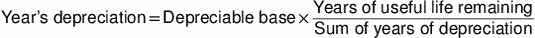##### CPA Exam For Dummies with Online PracticeHere are some important formulas that often appear on the CPA exam. Understanding these formulas will help you boost your test score. These formulas are used on nearly every CPA exam, so it's an easy way to get some correct answers under your belt. Also, knowing these formulas helps you understand other accounting concepts that are frequently tested.

Here is the journal entry to record a \$1,000 customer deposit. A deposit is a payment you receive before a product or service is delivered.

Debit (increase) cash \$1,000, credit (increase) unearned revenue \$1,000

Unearned revenue is a liability account. The customer deposit must be returned to the client if the product or service is not delivered. As a result, unearned revenue is a type of liability. When the product or service is delivered, you make this entry to recognize revenue:

Debit (reduce) unearned revenue \$1,000, credit (increase) revenue \$1,000

## Ending inventory formula

For many businesses, inventory is the largest asset category on the balance sheet. CPAs need to know how inventory costs flow through a business. This formula for ending inventory should be on every exam:

Ending inventory = Beginning inventory + Purchases – Cost of sales

## Retained earnings formula

The balance in retained earnings represents all the profit that a company has generated — and not distributed to owners — since inception. Exam candidates often misunderstand the activity in this account, so knowing this formula is important:

Retained earnings = Beginning retained earnings + Net income – Dividends

A net loss reduces retained earnings. Several other transactions may affect retained earnings, but this basic formula is the one that's tested most often.

## Profit on sale of inventory item: FIFO method

The first-in-first-out (FIFO) method of inventory valuation assumes that the oldest items are sold first. Because prices generally rise over time, the oldest items in inventory tend to be the cheapest.

Suppose that an item has a sale price of \$10,000 per unit. Here are the three items in inventory as of March 1. Each item includes the purchase date and the item's cost:

January 15 \$7,800 purchase, February 1 \$8,200 item, February 15 \$8,800 item

Say that one item is sold on March 15. Using the FIFO method, the company assumes that the oldest item (January 15) is sold. The profit on the sale is \$10,000 sale price – \$7,800 cost = \$2,200 profit.

## Profit on sale of inventory item: LIFO method

The last-in-first-out (LIFO) method of inventory valuation assumes that the newest items are sold first. Because prices generally rise over time, the newest items in inventory tend to be the most expensive.

Suppose the sale price is still \$10,000 per unit, and the same three items are in inventory as of March 1:

January 15 \$7,800 purchase, February 1 \$8,200 item, February 15 \$8,800 item

Say that one item is sold on March 15. Using the LIFO method, the company assumes that the newest item (February 15) is sold. The profit on the sale is \$10,000 sale price – \$8,800 cost = \$1,200 profit.

## Checking out stock option gains

Stock options are a very common way to reward employees for helping a company succeed over the long term. Because stock options are used frequently, the details are tested on the CPA exam.

A stock option enables the holder to buy common stock as a set price (called the strike price or exercise price) for a specific period of time. Stock options are often granted to key employees as a reward for staying at a company and helping to increase profitability. Here's a basic formula:

Stock option profit per share = Market price of stock per share – Exercise price of stock (buyer's cost) per share

In many cases, the employee's ownership of the stock options becomes vested, or earned over time. Suppose a manager must stay at the firm for two years to become fully vested. He or she will be vested for half of the stock options after one year and vested for all the options after two years.

Suppose that Sally is vested in stock options that allow her to buy 100 shares at a price of \$20 per share. Sally holds the options until the market price of the stock is \$40 per share. Sally decides to exercise the options, which means that Sally buys the stock shares at the strike price of \$20 and sells them at the current market price of \$40. Sally's gain is \$40 – \$20 = \$20 × 100 shares = \$2,000.

## Going over contribution margin

Contribution margin reveals the amount of revenue remaining to pay for fixed costs:

Contribution margin = Sales – Variable costs

Contribution margin is a useful tool for managers. After paying for fixed costs, any balance left is your profit. Keep in mind that contribution margin is not net income. Contribution margin, however, does give you some idea of your profitability.

Say, for example, that you manufacture baseball gloves. You sell the gloves for \$80 each, and your variable costs to make a glove total \$50 per unit. Variable costs typically include direct material costs (leather, in this case) and direct labor. Your contribution margin per unit is \$80 – \$50 = \$30. For each glove, you have \$30 left to cover fixed costs. Any amount left after paying your fixed costs will be profit.

## Using sum of the year's digits depreciation

Sum of the year's digit's depreciation (SYD) is an accelerated method of depreciation. Accelerated depreciation methods recognize more depreciation in the early years and less in later years. Keep in mind, however, that the total amount of be depreciated is the same, regardless of the depreciation method you choose. If, for example, you buy a truck for \$36,000 with \$6,000 in salvage value, you'll recognize \$30,000 in depreciation. If you recognize more depreciation in the early years, you'll recognize less depreciation in later years.

Suppose that you're going to depreciate that truck with a \$36,000 cost and a salvage value of \$6,000. Salvage value is the amount you can sell truck for at the end of its useful life. Your depreciable base is

Depreciable base = Cost – Salvage value

In this example, the depreciable base is \$36,000 cost – \$6,000 salvage value = \$30,000. The useful life of the truck is 5 years.

You calculate SYD depreciation using a fraction. The denominator of the fraction is the sum of each year of depreciation, and the numerator of the formula is the number of years of useful life remaining at the beginning of a particular year. You find a particular year's depreciation by multiplying this fraction by the depreciable base:In this case, the denominator is 1 + 2 + 3 + 4 + 5 = 15. At the beginning of the first year, 5 years of useful life remain. The fraction for Year 1 depreciation is 5 ÷ 15, or one-third. Depreciation in Year 1 is \$30,000 depreciable base × (5 ÷ 15) = \$10,000 depreciation. The fractional part of the Year 2 depreciation formula is 4 ÷ 15.